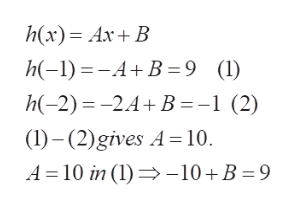# Write a rule for a linear function y = h(x), given that h (-1) = 9 and h (-2)= -1. Write your anwer in simplest form.

Question
15 views

Write a rule for a linear function y = h(x), given that h (-1) = 9 and h (-2)= -1. Write your anwer in simplest form.

check_circle

Step 1

To determine the expression h(x) =Ax+B (linear function, first degree) satisfying the given conditions

Step 2

Solve for A and B , using t...help_outlineImage Transcriptioncloseh(x) AxB h(-1)AB=9 (1) h(-2) 2.4B=-1 (2) (1)-(2)gives A 10 A 10 in (1 -10B 9 fullscreen

### Want to see the full answer?

See Solution

#### Want to see this answer and more?

Solutions are written by subject experts who are available 24/7. Questions are typically answered within 1 hour.*

See Solution
*Response times may vary by subject and question.
Tagged in

### Equations and In-equations